• 假定某个局部区域细胞识别中正常P(w1)和异常P（w2)两类先验概率分别为P(w1)=0.9, P(w2)=0.1现有一系列待观察的细胞，其观察值为：-2.67 -3.55 -1.24 -0.98 -0.79 -...依据最小错误率的贝叶斯决策对观察的结果进行分类。
• 最小错误率贝叶斯决策和最小风险贝叶斯决策matlab代码，贝叶斯决策理论方法，是统计模式识别中的一个基本方法。贝叶斯决策判据，既考虑了各类参考总体出现的概率大小，又考虑了因误判造成的损失大小，判别能力强。
• 基于最小错误率的贝叶斯手写数字分类器，包括注释，很详尽，是自己按照定义写的，训练集和测试集是用的mnist数据集，最终正确率为73.89%
• 计算男女身高,非常强大的matlab编程实现贝叶斯程序，可直接使用，模式识别
• 模式识别实例：包括最小错误率、最小风险贝叶斯决策matlab代码，注释清楚丰富。
• 含有三份代码，都是用matlab实现，分别是基于贝叶斯，基于朴素贝叶斯，基于最小错误率贝叶斯的手写数字识别代码。其中朴素贝叶斯用到了PCA，准确率达到95%
• 用FAMALE.TXT和MALE.TXT的数据作为训练样本集，建立Bayes分类器，用测试样本数据对该分类器进行测试。实验程序加报告加数据
• 两个贝叶斯分类器的例子，分别基于最小错误率与最小风险贝叶斯分类器
•贝叶斯分类器
• 最小错误率贝叶斯分类器（包含matlab程序）1. 最小错误贝叶斯分类器原理2.最小错误率贝叶斯分类器的图形表示3.三类最小错误率贝叶斯分类器实验实验代码 1. 最小错误贝叶斯分类器原理 在对模式进行识别时，在存在...


最小错误率贝叶斯分类器（包含matlab程序）
1. 最小错误贝叶斯分类器原理2.最小错误率贝叶斯分类器的图形表示3.三类最小错误率贝叶斯分类器实验实验代码

1. 最小错误贝叶斯分类器原理
在对模式进行识别时，在存在模棱两可的情况下，任何决策都存在判别错误的可能性。最小错误贝叶斯决策就是以错误率为自小的分类规则。使用p(e)表示错误概率，那么最小错误贝叶斯分类器的目的就是minp(e).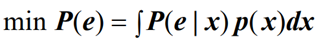对于两种模式，其中：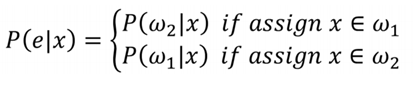即x属于第一类，判别为第二类的概率，x属于第二类，判别为第一类的概率。 可以看出使错误率最小的决策就是使后验概率最大的决策，因此最小错误率贝叶斯决策可写为：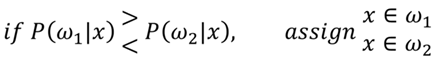其中，后验概率的计算使用贝叶斯公式计算，因此要已知先验概率p(ωi)以及类条件概率密度p(x|wi)。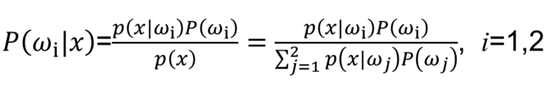判决公式还有很多等价公式。
2.最小错误率贝叶斯分类器的图形表示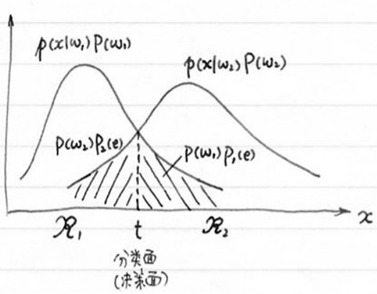错误率：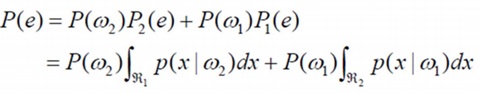对于多类问题的决策，思路跟两类问题类似，不同的只是计算量加重了许多；可以把每一类的后验概率当做是该类的一个判别函数gi(x),决策的过程就是各类的判别函数进行比较，最后取最大的那个。使用图形表示为：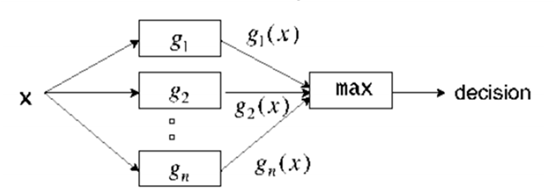3.三类最小错误率贝叶斯分类器实验
（1）使用函数产生mvnrnd三类服从高斯分布的样本点。高斯函数的参数作为输入参数输入，包括均值，协方差，个数，先验概率。 （2）为了区分三类样本，第一类使用蓝色，第二类使用绿色，第三类使用红色。 第一类错分的点使用黑色三角表示下来，第二类错分的店使用黄色+表示下来，第三类使用粉红色正方形表示出来。 （3）使用函数mvnpdf()实现高斯密度函数值的计算 （4）比较高斯密度值的大小，选取最大的一类，分到那一类。
输入参数分别为：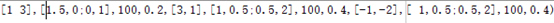实验结果：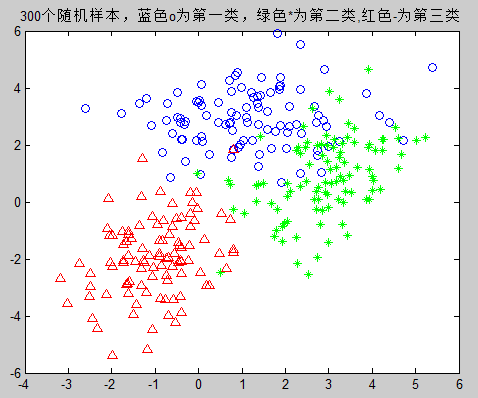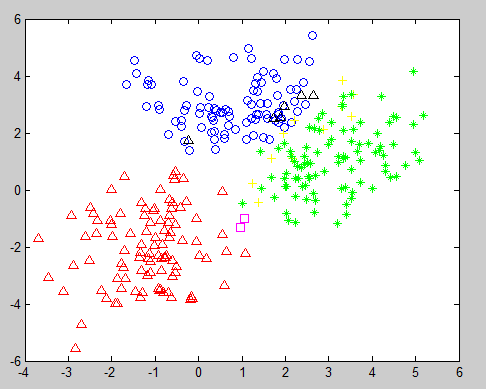实验代码
function self_mvnrnd1(varargin)%可自定义参数的函数
if(nargin==12)%判定输入参数是否为12
w1=mvnrnd(varargin{1},varargin{2},varargin{3});%第一类高斯函数密度值
w2=mvnrnd(varargin{5},varargin{6},varargin{7});%第二类
w3=mvnrnd(varargin{9},varargin{10},varargin{11});
figure(1);
plot(w1(:,1),w1(:,2),'bo');%蓝色o为第一类
hold on
plot(w2(:,1),w2(:,2),'g*');%绿色*为第二类
hold on
plot(w3(:,1),w3(:,2),'r^')
title('300个随机样本，蓝色o为第一类，绿色*为第二类,红色-为第三类');
w=[w1;w2;w3];
n1=0;%第一类正确个数
n2=0;%第二类正确个数
n3=0;%第三类正确的个数
figure(2);
%贝叶斯分类器
for i=1:(varargin{3}+varargin{7}+varargin{11})
x=w(i,1);
y=w(i,2);
g1=mvnpdf([x,y],varargin{1},varargin{2})*varargin{4};
g2=mvnpdf([x,y],varargin{5},varargin{6})*varargin{8};
g3=mvnpdf([x,y],varargin{9},varargin{10})*varargin{12};
if g1>g3&&g1>g2
if 1<=i&&i<=varargin{3}
n1=n1+1;%第一类正确个数
plot(x,y,'bo');%蓝色o表示正确分为第一类的样本
hold on;
else
plot(x,y,'k^');%红色的上三角形表示第一类错误分为第二类
hold on;
end
end
if g2>g3&&g2>g1
if varargin{3}<=i&&i<=(varargin{3}+varargin{7})
n2=n2+1;%第一类正确个数
plot(x,y,'g*');%绿色o表示正确分为第二类的样本
hold on;
else
plot(x,y,'y+');%红色的上三角形表示第一类错误分为第二类
hold on;
end
end
if g3>g1&&g3>g2
if (varargin{3}+varargin{7})<=i&&i<=(+varargin{7}+varargin{3}+varargin{11})
n3=n3+1;%第一类正确个数
plot(x,y,'r^');%绿色o表示正确分为第二类的样本
hold on;
else
plot(x,y,'ms');%红色的上三角形表示第一类错误分为第二类
hold on;
end
end
end
r1_rate=0;
r2_rate=0;
r3_rate=0;
r1_rate=n1/varargin{3}
r2_rate=n2/varargin{7}
r3_rate=n3/varargin{11}
print(r1_rate)
print(r2_rate)
print(r3_rate)
end
%%%%%%%%%
main.m
self_mvnrnd1([1 3],[1.5,0;0,1],100,0.2,[3,1],[1,0.5;0.5,2],100,0.4,[-1,-2],[ 1,0.5;0.5,2],100,0.4)

/
展开全文分类器 matlab
• 自己编写的，很实用的基于最小错误率的贝叶斯决策！
• 基于最小错误率的Bayes分类器的MATLAB实现，程序能出判别矩阵，训练样本分布图，测试样本分布图及分类后的分布图，可以选择训练样本数目，绝对能用！！
• 三次作业，分别用最小错误率贝叶斯分类器，Fisher线性分类器，人工神经网络进行识别。都采用matlab编程，前两种可在平台上手写数字并识别，人工神经网络版本的没有手写平台，能够通过程序读取图片并返回识别出的数字...
• 运用贝叶斯最小错误率法则识别手写数字，直接运行即可手写数字，然后识别。
• 基于最小错误率的贝叶斯决策实现手写数字识别1、实验目的2、实验方法及步骤（1）平台搭建（2）特征描述（3）建立最小错误率贝叶斯决策分类器（4）实现手写数字识别3、实验结果4、实验结果讨论5、实验结论 ...


基于最小错误率的贝叶斯决策实现手写数字识别
1、实验目的2、实验方法及步骤（1）平台搭建（2）特征描述（3）建立最小错误率贝叶斯决策分类器（4）实现手写数字识别

3、实验结果4、实验结果讨论5、实验结论文章代码链接：https://pan.baidu.com/s/1xgDBD8rUdDSi7Z7yozfaeA由于精力有限，关于其他方法的手写数字识别就没有写文章介绍。如需要其他方法的代码可私信我获取。

1、实验目的
本次试验的目的是建立一个手写数字识别系统，能够准确 的提取用户在手写板上写出的数字，并且能够正确地识别出来。
2、实验方法及步骤
此次实验选择MATLAB中的GUI界面来构建实验的平台框架，然后采用基于最小错误率的贝叶斯决策来作为识别方法，对手写数字进行识别。需要在GUI界面中搭建好所需的对象，并写入完成相关功能的程序。其实现的功能是首先能够识别用户输入的手写数字，并提取输入数字的特征；然后将得到的手写数字特征加上对应数字的标签，将其存入样本库中，用于后面手写数字的对比识别；最后根据用户输入的手写数字，提取特征并在样本库中根据贝叶斯决策来判断手写数字的类型，最后显示识别结果。主要分为以下四个步骤：
（1）平台搭建
本次手写数字识别系统的编程环境为MATLAB，通过在MATLAB的GUI界面中搭建框架，并在对应的对象的回调函数中编写相关的程序来实现手写数字识别的功能。   在GUI界面中需要的对象有两个静态文本框、一个坐标区、一个可编辑文本，搭建的界面及其功能如图1所示。图1 手写数字识别系统GUI界面

由图可以看出每个对象都有各自的的功能：“手写板”坐标用于用户书写数字；“特征提取”可编辑文本用于显示手写数字的每个特征值，以便后面的对比识别；“清除”按钮可以清除用户写在“手写板”上的数字以及“特征提取”中数字特征值；“提取特征”按钮的功能是对“手写板”中的数字进行识别处理，提取相应的特征值并输到“特征提取”可编辑文本中显示；“保存为样本”按钮则是用于建立样本数据库，将前期的样本特征保存到样本库中，以便后面数字的识别；而“识别”按钮则是根据当前输入的手写数字特征，在样本库中寻找对应的数字类型。
此次手写数字识别系统的平台搭建就如图1所示，然后通过搭建的界面根据需要实现的功能对相应的对象的回调函数编写程序。

（2）特征描述
特征描述就是对手写数字进行分析处理，得到数字的特征，然后根据每类数字各自的特征值来识别手写数字的类型。 在此次设计中，手写数字特征描述的步骤为：首先将含有全部特征信息的手写数字图像从坐标轴中提取出来，将提取出来的书写数字图像进行二值化处理；然后将处理后的图像分成

6

×

6

6\times6

小格，每个网格中全部1值像素点个数与全部像素点个数之比就是手写数字在这一网格中的特征值，得到36个网格中的特征值并存入特征矩阵feature中，即feature矩阵包含了手写数字所有的特征信息；最后显示手写数字的特征图，判断每个网格的特征值是否大于预先设定好的阈值（此次设计中取0.1），若大于阈值，则此网格全部像素点的值为1，反之网格全部像素点的值为0。其实现如图2所示。图2 手写数字特征显示

（3）建立最小错误率贝叶斯决策分类器
①分类器原理   对于具有多个特征参数的样本（本次设计samplelib数据样本d=36有个参数），其正态分布的概率密度函数可定义为

p

(

x

)

=

1

(

2

π

)

d

2

∣

Σ

∣

1

2

exp

⁡

{

−

1

2

(

x

−

μ

)

Σ

−

1

(

x

−

μ

)

T

}

p(\textbf{x})=\frac{1}{(2\pi)^{\frac{d}{2}}|\Sigma|^{\frac{1}{2}}}\exp\{-\frac{1}{2}(\textbf{x}-\mathbf{\mu})\Sigma^{-1}(\textbf{x}-\mathbf{\mu})^{T}\}

式中，

x

=

[

x

1

,

x

2

,

⋯

,

x

d

]

\textbf{x}=[x_{1},x_{2},\cdots,x_{d}]

是d维待识别样本特征向量，

μ

=

[

μ

1

,

μ

2

,

⋯

,

μ

d

]

\mathbf{\mu}=[\mu_{1},\mu_{2},\cdots,\mu_{d}]

是d维样本平均特征向量，

Σ

\Sigma

是

d

×

d

d\times d

维协方差矩阵。   本次设计采用最小错误率的贝叶斯决策，选择判别函数如下：

g

i

(

x

)

=

p

(

x

∣

w

i

)

⋅

p

(

w

i

)

,

i

=

1

,

2

,

⋯

,

10

g_{i}(\textbf{x})=p(\textbf{x}|w_{i})\cdot p(w_{i}),\ \ \ i=1,2,\cdots,10

式中，

p

(

w

i

)

p(w_{i})

为类别发生的先验概率，

p

(

x

∣

w

i

)

p(\textbf{x}|w_{i})

为类别的

w

i

w_{i}

类条件概率密度函数。   由判别规则，如果使

g

i

(

x

)

>

g

j

(

x

)

g_{i}(\textbf{x})>g_{j}(\textbf{x})

对一切

i

≠

j

i\neq j

成立，则将

x

\textbf{x}

归为

w

i

w_{i}

类。假设类条件概率密度函数

p

(

x

∣

w

i

)

p(\textbf{x}|w_{i})

服从正态分布，即有

p

(

x

∣

w

i

)

∼

N

(

μ

i

,

Σ

i

)

,

p(\textbf{x}|w_{i})\sim N(\mu_{i},\Sigma_{i}),

，那么上式就可以写为

g

i

(

x

)

=

p

(

w

i

)

(

2

π

)

d

2

∣

Σ

∣

1

2

exp

⁡

{

−

1

2

(

x

−

μ

)

Σ

−

1

(

x

−

μ

)

T

}

,

i

=

1

,

2

,

⋯

,

10

g_{i}(\textbf{x})=\frac{p(w_{i})}{(2\pi)^{\frac{d}{2}}|\Sigma|^{\frac{1}{2}}}\exp\{-\frac{1}{2}(\textbf{x}-\mathbf{\mu})\Sigma^{-1}(\textbf{x}-\mathbf{\mu})^{T}\},i=1,2,\cdots,10

对上式右端取对数，可得

g

i

(

x

)

=

−

1

2

(

x

−

μ

i

)

Σ

−

1

(

x

−

μ

i

)

T

+

ln

⁡

p

(

w

i

)

−

1

2

ln

⁡

∣

Σ

i

∣

−

d

2

ln

⁡

(

2

π

)

g_{i}(\textbf{x})=-\frac{1}{2}(\textbf{x}-\mathbf{\mu_{i}})\Sigma^{-1}(\textbf{x}-\mathbf{\mu_{i}})^{T}+\ln p(w_{i})-\frac{1}{2}\ln |\Sigma_{i}|-\frac{d}{2}\ln(2\pi)

上式中的第四项与样本所属类别无关，将其从判别函数中消去，不会改变分类结果，则判别函数

g

i

(

x

)

g_{i}(\textbf{x})

可简化为以下形式

g

i

(

x

)

=

−

1

2

(

x

−

μ

i

)

Σ

−

1

(

x

−

μ

i

)

T

+

ln

⁡

p

(

w

i

)

−

1

2

ln

⁡

∣

Σ

i

∣

g_{i}(\textbf{x})=-\frac{1}{2}(\textbf{x}-\mathbf{\mu_{i}})\Sigma^{-1}(\textbf{x}-\mathbf{\mu_{i}})^{T}+\ln p(w_{i})-\frac{1}{2}\ln |\Sigma_{i}|

②分类实现步骤 1）从samplelib.mat文件中读取样本（每类样本个数为20）特征参数，求出特征参数均值

μ

i

\mu_{i}

，然后从文件feature.dat中读取待分类样本的特征参数

x

\textbf{x}

； 2）求出每类样本的协方差矩阵，逆矩阵以及对应的行列式； 3）由于每类样本的个数相同，故先验概率

p

(

w

i

)

=

0.1

p(w_{i})=0.1

，根据一二步骤可求出类条件概率

p

(

x

∣

w

i

)

p(\textbf{x}|w_{i})

。 4）将每类样本参数代入判别函数，求取最大值即得到分类。
（4）实现手写数字识别
根据上述设计，得到了手写数字识别系统。首先，通过输入样本得到样本库。此次实验中存入样本库samplelib.mat的每类样本的个数为20，选取的特征参数为

6

×

6

6\times 6

。其系统实现如图3所示。图３　手写数字识别系统的实现

3、实验结果
通过设计的手写数字识别系统，首先构建一个训练样本集。通过向手写板中输入数字，获取手写数字的特征，存入相应标签的样本库中。在此次设计中，样本库有0-9十个类别，而且每个类别中存入了20个样本，以供手写数字的识别。   构建好样本库后，输入每个类别的手写数字进行了重复地识别测试。经实验发现，本次设计的手写数字识别效果很好，基本可以识别出所有书写规范的数字，只是当书写不规范时会发生错误，但也有少部分类型的手写数字识别率不高，如手写数字8，就在书写很规范时也会有很高的错误率。   针对手写数字8识别率不高的情况，我对其进行了大量的重复实验，根据实验结果可以发现，当手写数字8识别错误时，总是将其识别为0或者6，很少识别为其他数字。其错误识别结果如图4，5所示。图４　错误识别为６图５　错误识别为０

4、实验结果讨论
针对上述手写数字8识别率不高的情况进行讨论发现，只有数字书写特别规范时才能正确识别，而当书写不太规范时就会错误地识别为6或者0。分析可知，手写数字8的特征图与6或者0的特征图较为相似，故当书写不规范时的数字特征会与数字6或者0的特征更为相似，从而导致识别错误。 根据以上分析可得，有以下两种方法来解决此问题： （1）增加类样本数   增加每类数字的样本数，使样本库包含尽可能多的数字形状，从而增大容错率，提高识别误差。 （2）提取更多的数字特征   在本次设计中，我将手写数字分成

6

×

6

6\times 6

个小块来提取数字特征。如果将数字分为更多的小块来提取特征，则得到的数字信息会更精确，使得识别效果更加理想。
5、实验结论
本次手写数字识别系统实在MATLAB中的GUI界面上实现的，决策分类器则是采用的基于最小错误率的贝叶斯决策。通过以上实验可知，采用基于最小错误率的贝叶斯决策分类较为准确，此手写数字识别系统有较好的识别性能，而且根据上述讨论，本手写数字识别系统还有改进提升的空间。
文章代码链接：https://pan.baidu.com/s/1xgDBD8rUdDSi7Z7yozfaeA
提取码：jr6o
由于精力有限，关于其他方法的手写数字识别就没有写文章介绍。如需要其他方法的代码可私信我获取。
展开全文贝叶斯 MATLAB代码 手写数字识别 图像二值化
• 最小错误率的贝叶斯分类实验和可运行源代码。可以让读者迅速掌握贝叶斯分类的原理。源代码可编译可运行。贝叶斯分类
•  但是最小错误率标准在某些决策中是不合理的。比如在癌细胞识别的决策过程中，我们对于不同错误的接受程度不同，因此不同错误的决策要乘上不同的权重，来衡量决策的风险，也就形成了最小风险决策。    从...
01 贝叶斯决策理论要解决的问题
根据已有数据对新的数据行分类

02 从一个经典的例子说起
问题：已知若干条鲈鱼和马哈鱼的长度信息，根于一条未知品种的鱼的长度，判断其是鲈鱼还是马哈鱼。

思路：引入随机变量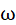,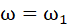：鲈鱼；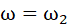：马哈鱼；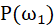：鲈鱼的概率;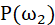：马哈鱼的概率。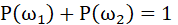；
根据已有两种鱼长度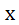的数据，我们可以知道类条件概率密度函数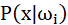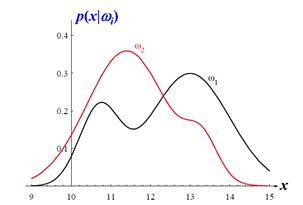那么可以根据贝叶斯公式

可以计算出后验概率密度函数，如下图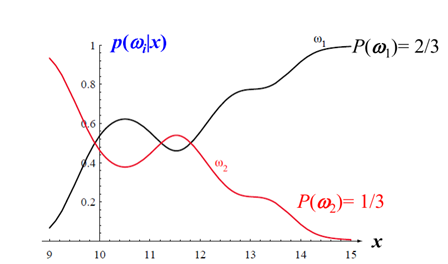03 最小错误率决策标准
给定鱼的长度，两类属性的概率一目了然，那么采取怎样的标准去判别呢？
最自然想到的标准便是 最小错误率 了
用数学表达就是

证明：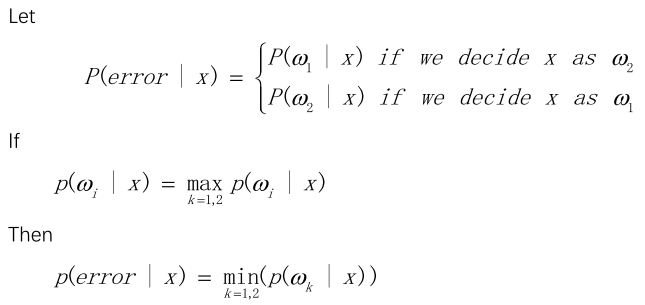启发式地证明：所谓概率最大就是取上图中上部分的曲线为决策分类，那么下部分的曲线就是错误决策分类，下部分曲线的概率自然是最小的。

04 最小风险决策标准
但是最小错误率标准在某些决策中是不合理的。比如在癌细胞识别的决策过程中，我们对于不同错误的接受程度不同，因此不同错误的决策要乘上不同的权重，来衡量决策的风险，也就形成了最小风险决策。

从例子入手：已知某个局部组织中有异常细胞，且正常细胞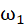和异常细胞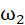的先验概率为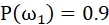和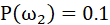。给一个待识别的细胞，其观测值为x, 从类条件概率密度函数中可查得：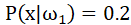，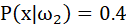。我们的风险如下表：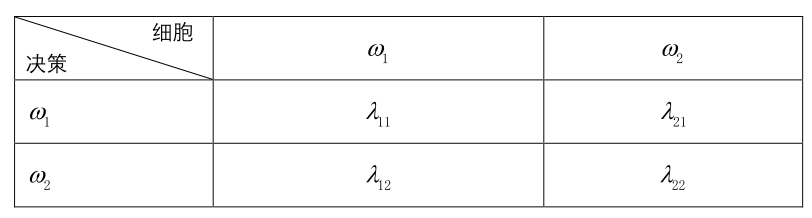其中：λij 表示第i类细胞被决策为j类的风险。显然 λij=0 ，因为这是正确决策。
此题中我们给定： λ=λ11,λ12,λ21,λ22=(0,6,1,0)
根据贝叶斯公式可得后验概率为

将x决策为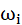的风险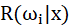为：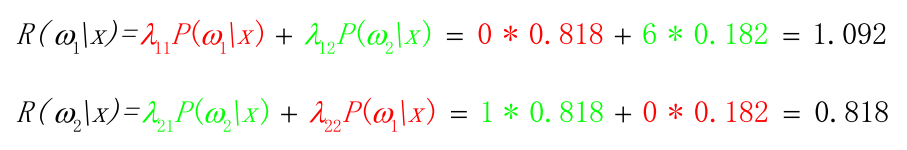红色部分为正确决策的风险，等于零，蓝色部分为分类错误的风险。
显然，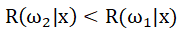，应该把x判定为异常细胞。
展开全文贝叶斯决策 模式分类
• 在用于分类的贝叶斯决策中，最优的条件可以是最小错误率或者最小风险。对连续变量： ，我们假定把它估计为： 所带来的损失函数为： 定义在样本x下的条件风险为： 则估计时总期望风险为： 现在的目标是对...
一、引言
1.用贝叶斯决策理论分类要事先知道两个条件及要求：
①.各类的先验概率：
及特征向量的条件概率密度：
或后验概率：
②.决策分类的类别一定
2.解决的问题：
已知一定数目的样本，设计分类器，对未知样本进行分类。
3.基于样本的两步贝叶斯决策
①首先根据样本估计和
记为和
②然后用估计的概率密度设计贝叶斯分类器
前提：训练样本的分布能代表样本的真实分布。每个样本集中的样本都是所谓独立同分布的随机变量，且有充分的训练样本
假设：当样本数N →∞时，如此得到的分类器收敛于理论上的最优解。 即满足：
→，→
4.先验概率与条件概率密度估计
①类的先验概率估计：可依靠经验或训练数据中各类出现的频率估计，交容易实现；
②类条件概率密度的估计：概率密度函数包含了一个随机变量的全部信息，估计起来比较困难。
5.概率密度估计的两种基本方法
①参数估计：根据对问题的一般性的认识，假设随机变量服从某种分布，分布函数的参数通过训练数据来估计。如：ML 估计，Bayesian估计
②非参数估计：不用模型，而只利用训练数据本身对概率密度做估计。如：Parzen窗法，kn-近邻估计法。
下面只着重介绍参数估计
二、最大似然估计与贝叶斯参数估计
1.最大似然估计基本原理
先做以下假设：
①估计的参数记为：。它是确定但未知的量（多个参数时为向量）；
②每类的样本集记作：，
其中样本都是从密度为的总体中独立抽取出来的，满足独立同分布条件；
③类条件概率密度具有某种确定的函数形式，只是其中的参数未知；
④各类样本只包含本类的分布信息，不同类别的参数是独立的，这样就可以分别对每一类单独处理。
现有以下样本：
有了以上假设，则获得以上样本的概率即出现样本中各个样本的联合概率是：

最大似然估计，通俗的理解，即为：参数为多少时观测值出现的概率最大。
最大似然估计量：
还可以定义对数似然函数：

2.最大似然估计的求解
①若待估参数为一维变量，即待估参数只有一个，其最大似然估计量就是如下微分方程的解：
或
当待估参数为多个未知参数组成的向量时，即：
求解似然函数的最大值就需要对该参数的每一维分别求导，即用下面的梯度算子：

或

3.正态分布下的最大似然估计
仅以单变量正态分布情况估计其均值与方差：
单变量正态分布如下：

从上述正太分布式可以得到：

分别对两个位置参数求偏导，得到：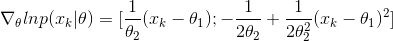最大似然估计应该是以下方程组的解：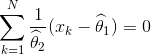与
解得：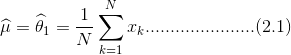ML估计总结：
①简单性
②收敛性：无偏或者渐近无偏
③如果假设的类条件概率模型正确，则通常能获得较好的结果。但果假设模型出现偏差，将导致非常差的估计结果。
4.贝叶斯估计基本原理
可以把概率密度函数的参数估计问题看作一个贝叶斯决策问题，但这里决策的不是离散类别，而是参数的值，是在连续空间里做的决策。
在用于分类的贝叶斯决策中，最优的条件可以是最小错误率或者最小风险。对连续变量：，我们假定把它估计为：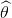所带来的损失函数为：
定义在样本x下的条件风险为：
则估计时总期望风险为：
现在的目标是对期望风险求最小，而条件风险都是非负的，求期望风险最小就等价于对所有可能的x求条件风险最小。在有限样本集合的情况下，我们所作的就是对所有的样本求条件风险最小，即：
在决策分类时，需要事先定义决策表即损失表，连续情况下需要定义损失函数，最常用的损失函数是平方误差损失函数，即：
可以证明，如果采用平方误差损失函数，则θ 的贝叶斯估计量θ*是在给定x 时θ 的条件期望，即：
在许多情况下 ,最小方差贝叶斯估计是最理想的，是贝叶斯的最优估计。
5.求贝叶斯估计的方法：（平方误差损失下）
①确定未知参数θ的先验分布密度 p(θ)
②求样本集的联合分布：
③利用贝叶斯公式求θ的 后验概率分布：
④求θ的贝叶斯估计量是：
6.正态分布时的贝叶斯估计
以最简单的一维正态分布模型为例来说明贝叶斯估计的应用。假设均值u为待估计参数，方差为已知。
均值u的先验分布也是正态分布，即：
求μ的后验概率：
分母只是用来对估计的后验概率进行归一化的常数项，可以暂时不考虑：

整理得：

由两式指数项中对应的系数相等得：
与
其中，
求解上述方程组得：

求μ的贝叶斯估计值：μ 的期望

①样本数目趋于无穷大时，第一项的系数趋于1，第二项系数趋于0，则估计的均值就是样本的算术平均，这与最大似然估计一致。
②当样本数目有限，若先验知识非常确定，则先验分布的方差就很小，则第一项系数就很小，第二项系数接近1，则估计主要由先验知识决定。
一般情况下，均值得贝叶斯估计是样本算术平均与先验分布均值之间进行加权平均。
总结：通过观察数据集X ,将先验概率密度P(θ) 转化为后验概率密度 P(θ|X) ，并期望其在真实的θ 值处有一个尖峰
7.ML估计和Bayesian估计的比较
①ML估计
1.参数为未知确定变量
2.没有利用参数先验信息
3.估计的概率模型与假设模型一致
4.可理解性好
5.计算简单
②Bayesian估计
1.参数为未知随机变量
2.利用参数的先验信息
3.估计的概率模型相比于假设模型会发生变化
4.可理解性差
5.计算复杂
三、分类器设计
1.常用概念
c类分类决策问题：按决策规则把d维特征空间分为 为c个决策区域。
决策面：划分决策域的边界面称为决策面，数学上用决策面方程表示。
判别函数：表达决策规则的函数，称为判别函数。
2.具体的判别函数、决策面方程、分类器设计
①定义一组判别函数：
根据决策规则：，即：
例如基于最小错误率贝叶斯判决规则，判别函数可定义为：

②决策面方程
类型w i 与w j 的 区域相邻，它们之间的决策面方程为：
一维特征空间的两个决策区域（d=1），决策面为分界点；
二维特征空间的两个决策区域（d=2），决策面为曲线；
三维特征空间，分界处是曲面；d维特征空间，分界处是超曲面。
3.正态分布下的最小错误率贝叶斯判别函数和决策面
1.判别函数：
其中：服从：

进行单调的对数变换，则判别函数为：

2.决策方程：

四、实验举例
1.实验内容
1.以身高为例，画出男女生身高的直方图并做对比；
2.采用最大似然估计方法，求男女生身高以及体重分布的参数；
3.采用贝叶斯估计方法，求男女生身高以及体重分布的参数（注明自己选定的参数情况）；
4.采用最小错误率贝叶斯决策，画出类别判定的决策面。并判断某样本的身高体重分别为(160,45)时应该属于男生还是女生？为(178,70)时呢？
2.具体步骤
1.通过python的xlrd包相应的库函数来读取excel文件中的数据，并将需要处理的数据存储与数组中，并通过matplotlib库函数画出男生和女生的身高分布直方图，如图一：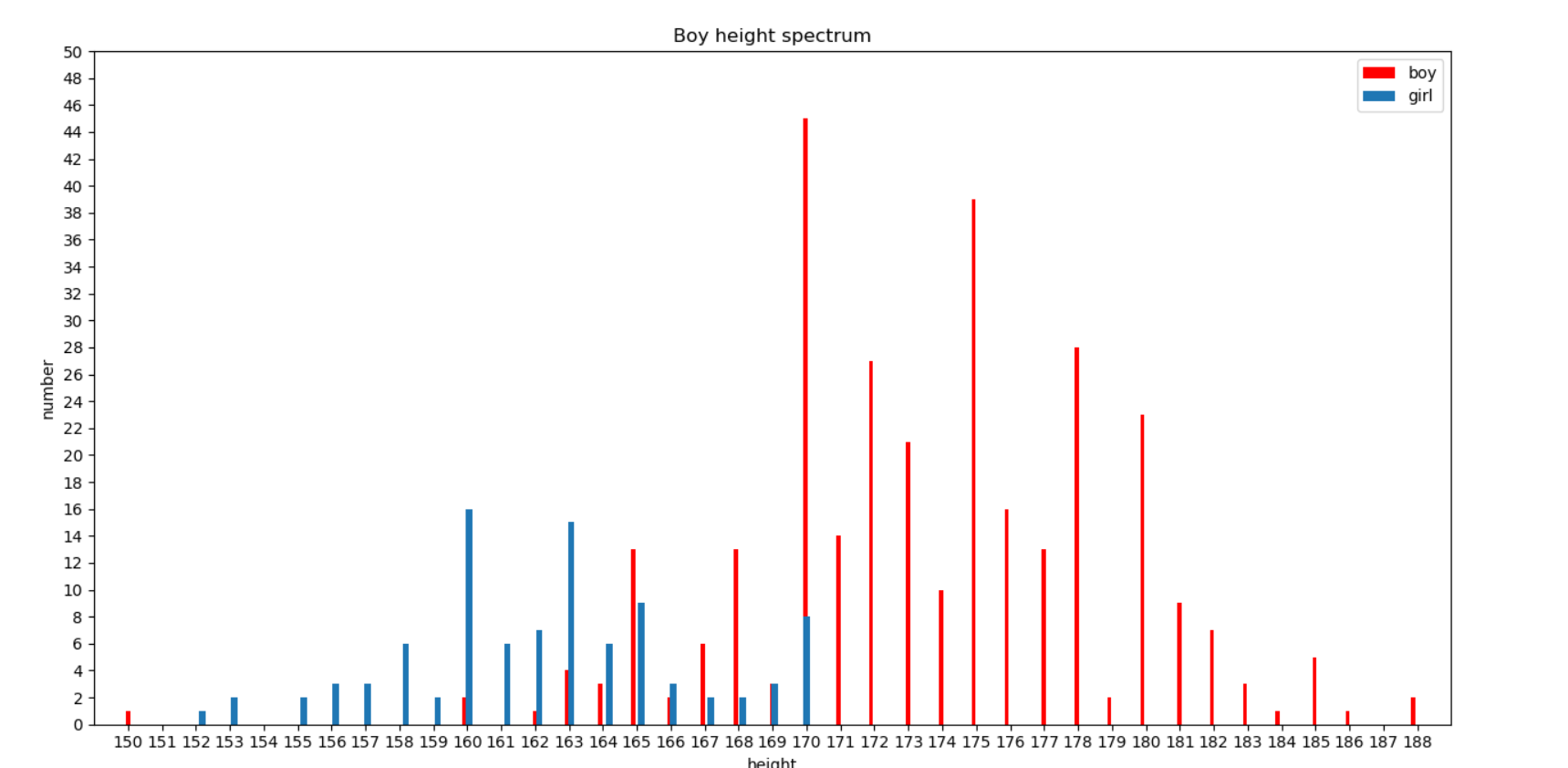图一
结果分析：从图中可以看，男女生的身高分布没有完全服从正态分布，主要由于样本数量比较少。很多时候，正态分布模型是一个合理假设。在特征空间中，某类样本较多分布在这类均值附近，远离均值的样本较少，一般用正态分布模型是合理的。在后续的实验分析中都将运用正态分布特性对男女生的样本进行分析。
2.采用最大似然估计方法，求男女生身高以及体重分布的参数；
假设身高体重相互独立并且都满足正态分布，采用最大似然法估计其参数，根据公式2.1、2.2求出男女生身高和体重的均值和方差。所求得的参数如表一所示：
女生身高  女生体重  男生身高  男生体重  均值(μ)  162.3125  50.7447  173.7388  66.0923  方差(σ2)  17.3606  21.1978  27.9827  86.5551
3.采用贝叶斯估计方法，求男女生身高以及体重分布的参数（假定方差已知，作业请注明自己选定的一些参数情况）。
特殊情况1:（先验知识可靠，样本不起作用），即
'''
特殊情况1:
variance0=0时，mean_bayes=mean0
先验知识可靠，样本不起作用
#以男生身高为例，选定参数：mean0=20,variance=10,variance0=0
'''
man_height_mean_bayes=get_mean_bayes(man_height,20,0,10)
print(man_height_mean_bayes)
 
结果为：20.0
特殊情况2：（先验知识十分不确定，完全依靠样本信息），即：
'''
特殊情况2:
variance0>>variance时，mean_bayes=sample_mean
先验知识十分不确定，完全依靠样本信息,结果与最大似然估计结果近似
#以女生身高为例，选定参数：mean0=50,variance=1,variance0=100
'''
woman_height_mean_bayes=get_mean_bayes(woman_height,50,100,1)
print(woman_height_mean_bayes)
 

结果为：162.3008019997917
4.采用最小错误率贝叶斯决策，画出类别判定的决策面。并判断某样本的身高体重分别为(160,45)时应该属于男生还是女生？为(178,70)时呢？
假设男女生身高及体重满足二维正态分布，记男生为w1，女生为w2，根据男女学生的人数比例确定先验概率: P(w1)=203/149=0.7658536585365854，P(w2)=1-P(w1)=0.23414634146341462
#求男生、女生先验概率
man_priori_probability=manlen/(manlen+womanlen)
woman_priori_probability=1-man_priori_probability
求男女生的协方差矩阵：
#①本题输入类数为2：即男生、女生；特征数为2：即身高、体重
#②求协方差矩阵
def get_covariance_matrix_coefficient(arr1,arr2):#arr1与arr2长度相等
datalength1=len(arr1)
datalength2=len(arr2)
sum_temp=[]
for i in range(datalength1):
sum_temp.append((arr1[i]-sum(arr1)/datalength1)*(arr2[i]-sum(arr2)/datalength2))
c12=sum(sum_temp)
covariance_matrix_c12=c12/(datalength1-1)
return covariance_matrix_c12
​
man_c11=man_height_variance
man_c22=man_weight_variance
man_c12=man_c21=get_covariance_matrix_coefficient(man_height,man_weight)
man_covariance_matrix=np.matrix([[man_c11,man_c12],[man_c21,man_c22]])
woman_c11=woman_height_variance
woman_c22=woman_weight_variance
woman_c12=woman_c21=get_covariance_matrix_coefficient(woman_height,woman_weight)
woman_covariance_matrix=np.matrix([[woman_c11,woman_c12],[woman_c21,woman_c22]])
求其决策方程：
# 定义等高线高度函数
def f(sample_height, sample_weight):
mytemp1=np.zeros(shape=(100,100))
for i in range(100):
for j in range(100):
sample_vector=np.matrix([[sample_height[i,j]],[sample_weight[i,j]]])
sample_vector_T=np.transpose(sample_vector)
#定义决策函数
mytemp1[i,j]=0.5*np.transpose(sample_vector-man_feature_mean_vector)*(np.linalg.inv(man_covariance_matrix))*\
(sample_vector-man_feature_mean_vector)-0.5*np.transpose(sample_vector-woman_feature_mean_vector)*\
(np.linalg.inv(woman_covariance_matrix))*(sample_vector-woman_feature_mean_vector)+\
0.5*math.log((np.linalg.det(man_covariance_matrix))/(np.linalg.det(woman_covariance_matrix)))-\
math.log(man_priori_probability/woman_priori_probability)
return mytemp1
使用python库函数plt.contour画类别决策面，并将(160,45)与(178,70)打点与图上，可知(160,45)属于女生，(178,70)属于男生。结果如图二。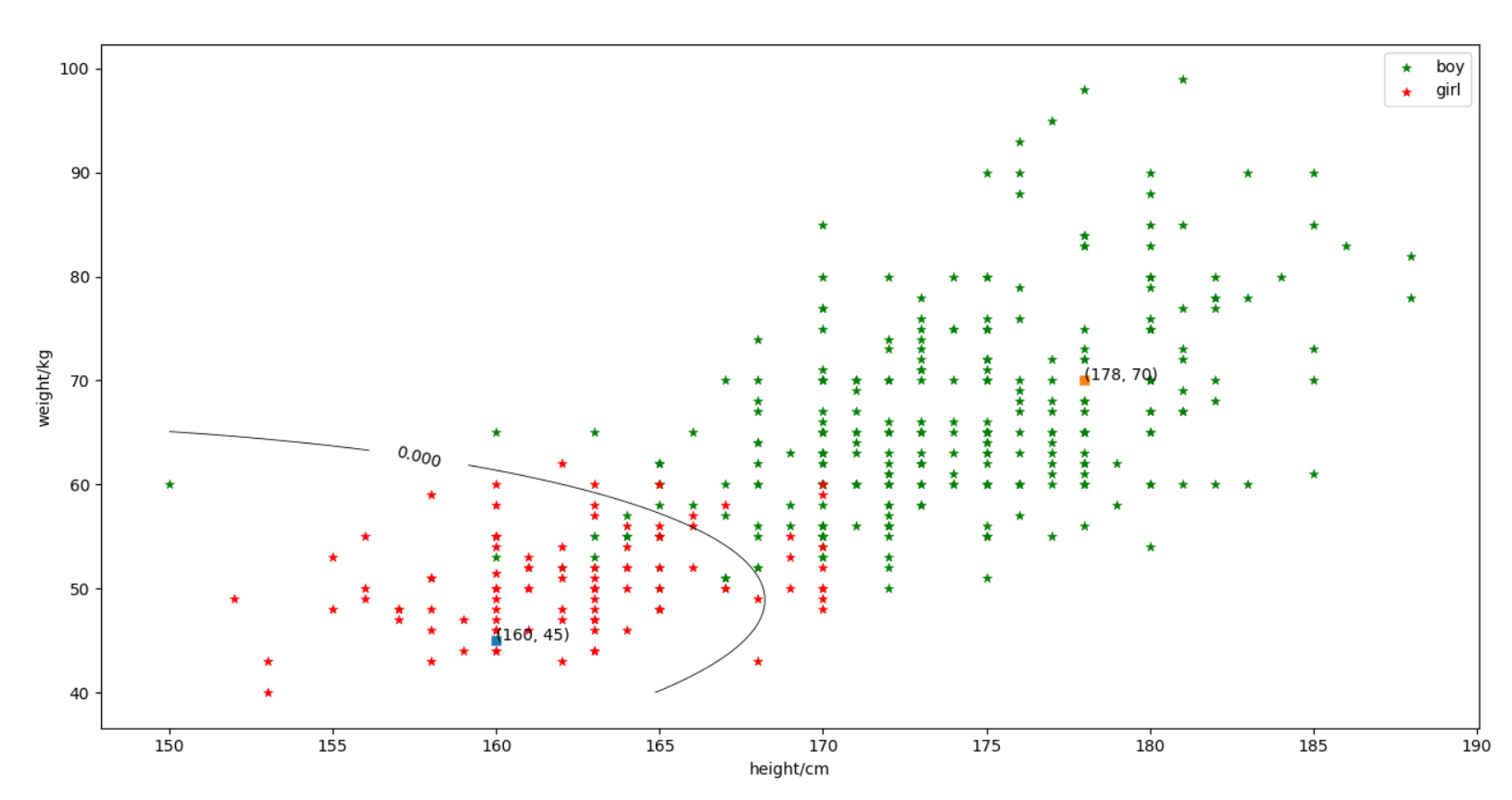完整代码（初版）
import xlrd
import math
import numpy as np
import scipy.stats as st
import matplotlib.pyplot as plt
from scipy.stats import norm

'''
Take the height as an example, draw a histogram of the
height of the boys and girls and compare
'''
mydata = xlrd.open_workbook('D:/program/py_code/data_2018.xls')
mysheet1 = mydata.sheet_by_name("Sheet1")

#获取行数、列数
nRows=mysheet1.nrows
nCols=mysheet1.ncols

#用于存取男生女生身高数据
man_height=[]
woman_height=[]

#获取第4列的内容:身高
for i in range(nRows):
if i+1<nRows:
if mysheet1.cell(i+1,1).value==1:
man_height.append(mysheet1.cell(i+1,3).value)
elif mysheet1.cell(i+1,1).value==0:
woman_height.append(mysheet1.cell(i+1,3).value)

#获取男、女生的数量
manlen=len(man_height)
womanlen=len(woman_height)

#画男女生身高频谱图
plt.hist(man_height,manlen,align='left',color='red',label='boy')
plt.hist(woman_height,womanlen,align='right',label='girl')
plt.legend(loc=0)
plt.xlabel('height')
plt.xlim(min(man_height+woman_height)-1,max(man_height+woman_height)+1)
plt.ylabel('number')
plt.title('Boy height spectrum')
#xsticks与yticks：指定坐标轴的刻度
plt.xticks(np.arange(min(man_height+woman_height),max(man_height+woman_height)+1,1.0))
plt.yticks(np.linspace(0,50,26))
plt.show()

'''
Using the maximum likelihood estimation method to find the
parameters of height and weight distribution for boys and girls
'''
#用于存取男生女生体重数据
man_weight=[]
woman_weight=[]

#将男女生体重数据从第5列中进行分离，并保存在上述空数组中
for i in range(nRows):
if i+1<nRows:
if mysheet1.cell(i+1,1).value==1:
man_weight.append(mysheet1.cell(i+1,4).value)
elif mysheet1.cell(i+1,1).value==0:
woman_weight.append(mysheet1.cell(i+1,4).value)
#fit(data):Return MLEs for shape, location, and scale parameters from data
#norm.fit(x)就是将x看成是某个norm分布的抽样，求出其最好的拟合参数（mean, std）
man_height_mean, man_height_std 	= norm.fit(man_height)#男生升高分布参数
man_weight_mean, man_weight_std 	= norm.fit(man_weight)#男生体重分布参数
woman_height_mean, woman_height_std = norm.fit(woman_height)#女生升高分布参数
woman_weight_mean, woman_weight_std = norm.fit(woman_weight)#女生体重分布参数

man_height_variance=man_height_std**2
man_weight_variance=man_weight_std**2
woman_height_variance=woman_height_std**2
woman_weight_variance=woman_weight_std**2
print(man_height_mean,man_height_variance,man_weight_mean,man_weight_variance)
print(woman_height_mean,woman_height_variance,woman_weight_mean,woman_weight_variance)

'''
采用贝叶斯估计方法，求男女生身高以及体重分布的参数（注明自己选定的参数情况）
'''
man_height_mean_cntr, man_height_var_cntr, man_height_std_cntr=st.bayes_mvs(man_height)
man_weight_mean_cntr, man_weight_var_cntr, man_weight_std_cntr=st.bayes_mvs(man_weight)
woman_height_mean_cntr, woman_height_var_cntr, woman_height_std_cntr=st.bayes_mvs(woman_height)
woman_weight_mean_cntr, woman_weight_var_cntr, woman_weight_std_cntr=st.bayes_mvs(woman_weight)
#print(man_height_mean_cntr.statistic,man_weight_mean_cntr.statistic)
#print(woman_height_mean_cntr.statistic,woman_weight_mean_cntr.statistic)

def get_mean_bayes(arr,mean0,variance0,variance):
datasum=sum(arr)
datalen=len(arr)
mean_bayes=(variance0*datasum+variance*mean0)/(datalen*variance0+variance)
return mean_bayes

'''
特殊情况1:
variance0=0时，mean_bayes=mean0
先验知识可靠，样本不起作用
#以男生身高为例，选定参数：mean0=20,variance=10,variance0=0
'''
man_height_mean_bayes=get_mean_bayes(man_height,20,0,10)
print(man_height_mean_bayes)

'''
特殊情况2:
variance0>>variance时，mean_bayes=sample_mean
先验知识十分不确定，完全依靠样本信息,结果与最大似然估计结果近似
#以女生身高为例，选定参数：mean0=50,variance=1,variance0=100
'''
woman_height_mean_bayes=get_mean_bayes(woman_height,50,100,1)
print(woman_height_mean_bayes)

'''
4.	采用最小错误率贝叶斯决策，画出类别判定的决策面。并判断某样本
的身高体重分别为(160,45)时应该属于男生还是女生？为(178,70)时呢？
'''
#①本题输入类数为2：即男生、女生；特征数为2：即身高、体重
#②求协方差矩阵
def get_covariance_matrix_coefficient(arr1,arr2):#arr1与arr2长度相等
datalength1=len(arr1)
datalength2=len(arr2)
sum_temp=[]
for i in range(datalength1):
sum_temp.append((arr1[i]-sum(arr1)/datalength1)*(arr2[i]-sum(arr2)/datalength2))
c12=sum(sum_temp)
covariance_matrix_c12=c12/(datalength1-1)
return covariance_matrix_c12

man_c11=man_height_variance
man_c22=man_weight_variance
man_c12=man_c21=get_covariance_matrix_coefficient(man_height,man_weight)
man_covariance_matrix=np.matrix([[man_c11,man_c12],[man_c21,man_c22]])
woman_c11=woman_height_variance
woman_c22=woman_weight_variance
woman_c12=woman_c21=get_covariance_matrix_coefficient(woman_height,woman_weight)
woman_covariance_matrix=np.matrix([[woman_c11,woman_c12],[woman_c21,woman_c22]])
print(woman_covariance_matrix)

#求男生、女生先验概率
man_priori_probability=manlen/(manlen+womanlen)
woman_priori_probability=1-man_priori_probability
#print(woman_priori_probability)

man_feature_mean_vector=np.matrix([[man_height_mean],[man_weight_mean]])
woman_feature_mean_vector=np.matrix([[woman_height_mean],[woman_weight_mean]])

# 定义等高线高度函数
def f(sample_height, sample_weight):
mytemp1=np.zeros(shape=(100,100))
for i in range(100):
for j in range(100):
sample_vector=np.matrix([[sample_height[i,j]],[sample_weight[i,j]]])
sample_vector_T=np.transpose(sample_vector)
#定义决策函数
mytemp1[i,j]=0.5*np.transpose(sample_vector-man_feature_mean_vector)*(np.linalg.inv(man_covariance_matrix))*\
(sample_vector-man_feature_mean_vector)-0.5*np.transpose(sample_vector-woman_feature_mean_vector)*\
(np.linalg.inv(woman_covariance_matrix))*(sample_vector-woman_feature_mean_vector)+\
0.5*math.log((np.linalg.det(man_covariance_matrix))/(np.linalg.det(woman_covariance_matrix)))-\
math.log(man_priori_probability/woman_priori_probability)
return mytemp1

sample_height = np.linspace(150, 180, 100)
sample_weight = np.linspace(40, 80, 100)
# 将原始数据变成网格数据
Sample_height, Sample_weight = np.meshgrid(sample_height, sample_weight)
# 填充颜色
plt.contourf(Sample_height, Sample_weight, f(Sample_height,Sample_weight), 0, alpha = 0)
# 绘制等高线,圈内为女生，圈外为男生
C = plt.contour(Sample_height, Sample_weight, f(Sample_height,Sample_weight), 0, colors = 'black',linewidths=0.6)
# 显示各等高线的数据标签
plt.clabel(C, inline = True, fontsize = 10)

#显示男女生样本散点图

p1=plt.scatter(man_height, man_weight,c='g', marker = '*',linewidths=0.4)
p2=plt.scatter(woman_height, woman_weight,c='r', marker = '*',linewidths=0.4)

# 定义显示坐标函数
def Display_coordinates(m, n):
plt.scatter(m, n, marker = 's',linewidths=0.4)
plt.annotate((m,n), xy = (m, n))
return

#并判断某样本的身高体重分别为(160,45)时应该属于男生还是女生？为(178,70)时呢
Display_coordinates(160,45)
Display_coordinates(178,70)
label=['boy','girl']
plt.legend([p1, p2],label,loc=0)
plt.xlabel('height/cm')
plt.ylabel('weight/kg')
plt.show()



展开全文模式识别 贝叶斯决策分类 python
• 最后，调用self_mvnrnd()函数可以用最小错误率贝叶斯分类器对其进行分类，统计正确分类的百分比，并在二维图上用不同的颜色画出正确分类和错分的样本。 一、 技术论述 二、 实验步骤及实验结果 ...贝叶斯分类器 matlab算法
• 其中女生类别标号为0，男生类别标号为1，数据集为dataset1.txt，dataset2.txt，dataset3.txt，第一列为身高，第二列为体重，第三列为类别标号分类 贝叶斯
• 1、贝叶斯公式 首先要知道贝叶斯公式： ...接下来阐释基于最小错误率的思想，以及贝叶斯公式在其中如何发挥作用。 在模式分类时，我们的目标是尽量减少分类的错误，即追求最小的错误率，用式子表达就是： 这个...
• 本代码给出了模式识别中贝叶斯决策方法的C++实现和matlab实现代码，对相关的学习具有引导作用。希望能够对你们的学习具有帮助！
• 理解掌握最小错误率贝叶斯分类器 2实验环境 Windows 10，Python 3.7.4，PyCharm 2019.2.3 3实验原理 Bayes公式：设实验E的样本空间为S，X为E的事件，w1，w2，...，wn为S的一个划分（即类别）且有P(X)>0，P...
• 最小错误率的贝叶斯决策】 贝叶斯定理： 以两类问题为例，已知两类别和的先验概率和 以及类别特征的条件概率和 现给定一个样本的特征x 分析其属于何种类别？ 判别函数： 若 则 X属于 若 则 X属于 ...
• matlab中洋红色代码设计最小错误率分类器 作者： 奈穆尔·哈克 F 1 目标 本实验的目的是使用高斯分布建模的后验概率对一些样本点进行分类，以计算似然概率。 这种分类器的目的是在分类过程中最小化错误率。 因此，该...
• 最小错误率的贝叶斯决策的理解： “输入x，判定为w1类，此判断错误的概率”等价于“输入x，判定为w2类,此判断正确的概率”机器学习
• 第22卷第5期122 2006年 5月农业工程学报TransactionsoftheCSAEV01．22No．5May2006基于最小错误率贝叶斯决策的苹果图像分割包晓敏，汪亚明(浙江理工大学信息电子...